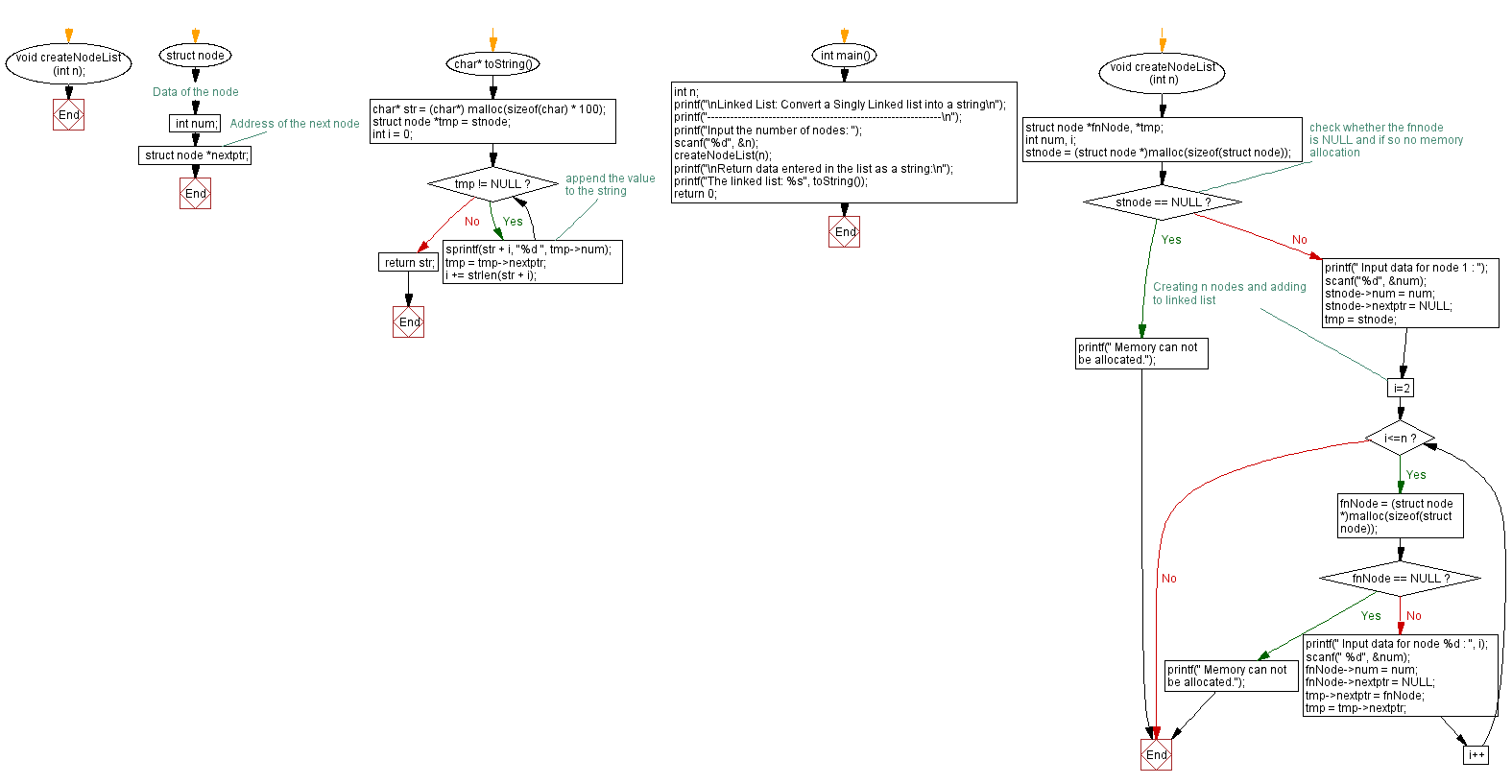﻿ C Program: Convert a Singly Linked list into a string

# C Exercises: Convert a Singly Linked list into a string

## C Singly Linked List : Exercise-11 with Solution

Write a C program to convert a Singly Linked list into a string and returns it.

Sample Solution:

C Code:

``````#include<stdio.h>
#include <stdlib.h>
#include <string.h>

struct node
{
int num;                        //Data of the node
struct node *nextptr;           //Address of the next node
}*stnode;

void createNodeList(int n);

char* toString() {
char* str = (char*) malloc(sizeof(char) * 100);
struct node *tmp = stnode;
int i = 0;
while(tmp != NULL) {
sprintf(str + i, "%d ", tmp->num); // append the value to the string
tmp = tmp->nextptr;
i += strlen(str + i);
}
return str;
}
int main()
{
int n;
printf("-------------------------------------------------------------\n");
printf("Input the number of nodes: ");
scanf("%d", &n);
createNodeList(n);
printf("\nReturn data entered in the list as a string:\n");
return 0;
}

void createNodeList(int n)
{
struct node *fnNode, *tmp;
int num, i;
stnode = (struct node *)malloc(sizeof(struct node));

if(stnode == NULL) //check whether the fnnode is NULL and if so no memory allocation
{
printf(" Memory can not be allocated.");
}
else
{
printf(" Input data for node 1 : ");
scanf("%d", &num);
stnode->num = num;
stnode->nextptr = NULL;
tmp = stnode;
for(i=2; i<=n; i++)
{
fnNode = (struct node *)malloc(sizeof(struct node));
if(fnNode == NULL)
{
printf(" Memory can not be allocated.");
break;
}
else
{
printf(" Input data for node %d : ", i);
scanf(" %d", &num);

fnNode->num = num;
fnNode->nextptr = NULL;

tmp->nextptr = fnNode;
tmp = tmp->nextptr;
}
}
}
}
```
```

Sample Output:

```
-------------------------------------------------------------
Input the number of nodes: 3
Input data for node 1 : 10
Input data for node 2 : 20
Input data for node 3 : 30

Return data entered in the list as a string:
The linked list: 10 20 30
```

Flowchart :C Programming Code Editor:

What is the difficulty level of this exercise?

﻿